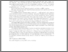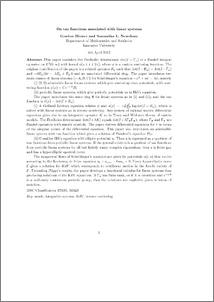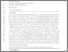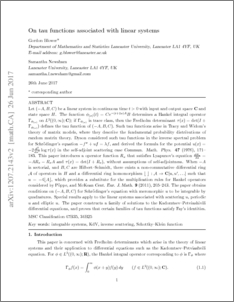# On tau functions associated with linear systems

Blower, Gordon and Newsham, Samantha (2012) On tau functions associated with linear systems. Working Paper. UNSPECIFIED. (Unpublished)Preview
PDF
dethankelshort.pdf - Submitted VersionPreview
PDF (Version 2 26/6/17)
1207.2143.pdf - Submitted Version

## Abstract

\noindent {\bf Abstract} This paper considers the Fredholm determinant $\det (I-\Gamma_x)$ of a Hankel integral operator on $L^2(0, \infty )$ with kernel $\phi (s+t+2x)$, where $\phi$ is a matrix scattering function. The original contribution of the paper is a related operator $R_x$ such that $\det (I-R_x)=\det (I-\Gamma_x)$ and $-dR_x/dx=AR_x+R_xA$ and an associated differential ring. The paper introduces two main classes of linear systems $(-A,B,C)$ for Schr\"odinger's equation $-\psi''+u\psi =\lambda \psi$, namely\par \indent (i) $(2,2)$-admissible linear linear systems which give scattering class potentials, with scattering function $\phi (x)=Ce^{-xA}B$;\par \indent (ii) periodic linear systems, which give periodic potentials as in Hill's equation.\par \indent The paper introduces the state ring ${\bf S}$ for linear systems as in (i) and (ii), and the tau function is $\tau (x) =\det (I+R_x)$. \par \indent (i) A Gelfand--Levitan equation relates $\phi$ and $u(x)=-2{{d^2}\over{dx^2}}\log\det (I-R_x)$, which is solved with linear systems as in inverse scattering. Any system of rational matrix differential equations gives rise to an integrable operator $K$ as in Tracy and Widom's theory of matrix models. The Fredholm determinant $\det (I+\lambda K)$ equals $\det (I+\lambda \Gamma_\Phi\Gamma_\Psi )$, where $\Gamma_\Phi$ and $\Gamma_\Psi$ are Hankel operators with matrix symbols. The paper derives differential equations for $\tau$ in terms of the singular points of the differential equation. This paper also introduces an admissible linear system with tau function which gives a solution of Painlev\'e's equation $P_{II}$.\par \indent (ii) Consider Hill's equation with elliptic potential $u$. Then $u$ is expressed as a quotient of tau functions from periodic linear systems. If the general solution is a quotient of tau functions from periodic linear systems for all but finitely many complex eigenvalues, then $u$ is finite gap and has a hyperelliptic spectral curve. The isospectral flows of Schr\"odinger's equation are given by potentials $u(t,x)$ that evolve according to the Korteweg de Vries equation $u_t+u_{xxx}-6uu_x=0.$ Every hyperelliptic curve ${\cal E}$ gives a solution for $KdV$ which corresponds to rectilinear motion in the Jacobi variety of ${\cal E}$. Extending P\"oppe's results, the paper develops a functional calculus for linear systems thus producing solutions of the KdV equations. If $\Gamma_x$ has finite rank, or if $A$ is invertible and $e^{-xA}$ is a uniformly continuous periodic group, then the solutions are explicitly given in terms of matrices.\par

Item Type:
Monograph (Working Paper)
Due to be submitted for publication in refereed mathematical journal.
Uncontrolled Keywords:
/dk/atira/pure/subjectarea/aacsb/disciplinebasedresearch
Subjects:
Departments:
ID Code:
53468
Deposited By:
Deposited On:
17 Apr 2012 08:16
Refereed?:
No
Published?:
Unpublished